# ISEE Upper Level Quantitative : How to add exponential variables

## Example Questions

### Example Question #1 : How To Add Exponential Variables

Simplify: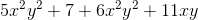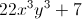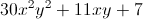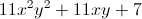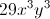The expression cannot be simplified furtherExplanation:

Group and combine like terms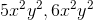: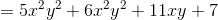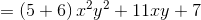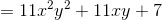### Example Question #2 : Variables And Exponents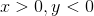Which is the greater quantity?

(a)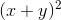(b)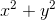(b) is greater.

(a) and (b) are equal.

(a) is greater.

It is impossible to tell from the information given.

(b) is greater.

Explanation: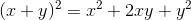Sinceandhave different signs,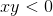, and, subsequently,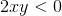Therefore,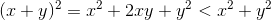This makes (b) the greater quantity.

### Example Question #3 : Variables And Exponents

Assume thatandare not both zero. Which is the greater quantity?

(a)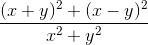(b)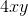It is impossible to tell from the information given.

(a) and (b) are equal.

(b) is greater.

(a) is greater.

It is impossible to tell from the information given.

Explanation:

Simplify the expression in (a):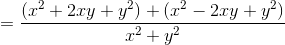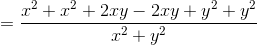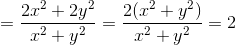Therefore, whether (a) or (b) is greater depends on the values ofand, neither of which are known.

### Example Question #4 : Variables And Exponents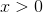Which is the greater quantity?

(a)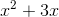(b)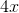(a) and (b) are equal

(b) is greater

It is impossible to tell from the information given

(a) is greater

It is impossible to tell from the information given

Explanation:

We give at least one positive value offor which (a) is greater and at least one positive value offor which (b) is greater.

Case 1:(a)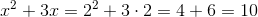(b)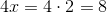Case 2: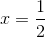(a)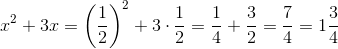(b)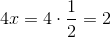Therefore, either (a) or (b) can be greater.

### Example Question #1 : How To Add Exponential Variables

Assume all variables to be nonzero.

Simplify: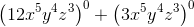None of the answer choices are correct.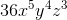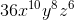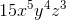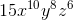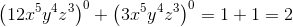.